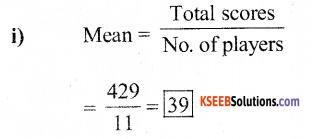# KSEEB Solutions for Class 7 Maths Chapter 3 Data Handling Ex 3.2

Students can Download Chapter 3 Data Handling Ex 3.2, Question and Answers, Notes Pdf, KSEEB Solutions for Class 7 Maths, Karnataka State Board Solutions help you to revise complete Syllabus and score more marks in your examinations.

## Karnataka State Syllabus Class 7 Maths Chapter 3 Data Handling Ex 3.2

Question 1.
The scores in mathematics test (out of 25) of 15 students is as follows :
19, 25, 23, 20, 9, 20,15,10, 5, 16, 25, 20, 24,12, 20
Find the mode and median of this data. Are they same?
Solution:
The scores in Mathematics test of 15 students should be arranged in ascending order.
5, 9, 10, 12, 15, 16, 19, 20, 20, 20, 20, 23, 24, 25, 25.
Median is the middle score Median = 20
Mode is the highest frequency of scores = 20
∴ Yes, the median and mode are the same.

Question 2.
The runs scored in a cricket match by 11 players is as follows :
6, 15, 120, 50, 100, 80, 10, 15, 8, 10, 15 Find the mean, mode, and median of this data. Are the three same?
Solution:
The runs scored in a cricket match is arranged in ascending order.
6, 8, 10, 10, 15. 15, 15, 50, 80, 100, 120ii) Median is the middle score. It is = 15

iii) Mode is the highest frequency score = 15
Mean, Median, and Mode is not the same.Question 3.
The weights (in kg.) of 15 students of a class are :
38, 42, 35, 37, 45, 50, 32, 43, 43, 40, 36, 38, 43, 38, 47
i) Find the mode and median of this data.
Solution:
The weight of 15 students is arranged in ascending order.
32, 35, 36, 37, 38, 38, 38, 40, 42, 43, 43, 45, 47, 50.
Median is the middle score = 40 kgs. m
Mode is the highest frequency score = 38 and 43.

ii) Is there more than one mode?
Solution:
Yes, here there are 2 modes.

Question 4.
Find the mode and median of the data : 13,16,12,14,19,12,14,13,14
Solution:
The given data is arranged in ascending order.
12, 12, 13, 13, 14, 14, 14, 16, 19
∴ The middle number is median = 14
∴ The mode is the highest frequency score = 14Question 5.
Tell whether the statement is true or false :
i) The mode is always one of the numbers in data.  True

ii) The mean is one of the numbers in data.  False

iii) The median is always one of the numbers in data.  True

iv) The data 6, 4, 3, 8, 9,12,13, 9 has mean 9.  False